# Dimensionless Analysis of the Temperature Field of Surrounding Rock in Coalface with a Finite Volume Method

Dimensionless Analysis of the Temperature Field of Surrounding Rock in Coalface with a Finite Volume Method

Yueping Qin Song KongWei Liu Jiansong Wu Huaitao Song

School of Resources and Safety Engineering, China University of Mining and Technology (Beijing), Beijing 100083, China

Corresponding Author Email:
phoenixks@live.cn
Page:
151-157
|
DOI:
http://doi.org/10.18280/ijht.330323
N/A
|
Accepted:
N/A
|
Published:
30 September 2015
| Citation

OPEN ACCESS

Abstract:

The thermal conductive mathematical model of surrounding rock was established in a moving coordinate in order to determine the temperature field of surrounding rock around coalface with a moving boundary. It was made possible to have a non-dimensional mathematical model by introducing dimensionless parameters such as Biot coefficient and Peclet coefficient. The dimensionless mathematical model was dispersed with a finite volume method, and then a computer code was developed for determining the temperature field of surrounding rock in coalface. Under the condition where the ratio of face width and semi mining height i s 8/3  for example, the dimensionless temperature distribution of surrounding rock is obtained by assuming both the Biot coefficient and Peclet coefficient to be parallel. When the Biot coefficient and Peclet coefficient are assumed to be variants, the results r eveal that the temperature increases with the increase of Peclet coefficient at a constant Biot coefficient, while  the  temperature  decreases  with the increase of Biot coefficient at a constant Peclet coefficient. Five kinds of working face are chosen for calculation  with typical ratio of face width and semi mining height, and each variation curves of unstable heat transfer  criterion with increasing  Peclet coefficient are obtained with different values of the Biot coefficient, which reveals that the unstable heat transfer criterion increases with the increase of Biot coefficient and Peclet coefficient. The complex calculation of heat dissipating capacity is greatly simplified and the results are given universal significance. The unstable heat transfer criterion can be determined by  a consulting graph, and this method provides critical parameters for forecasting air temperature and calculating chilling requirements  in coalface.

Keywords:

Coalface, Moving coordinate, Temperature field of surrounding rock, Dimensionless analysis, Finite volume method

1. Introduction

The underground thermal environment impedes the effective exploitation of deep resources in mines. The inhibiting effect becomes increasingly apparent with the increase of mining depth and degree of mechanization. The climatic conditions of high temperatures and humidity threaten the health of workers and hamper the safe production in mines. The thermal equilibrium of the human body is likely destabilized in the thermal environment, resulting in a decrease in production efficiency and an increase in the accident rate . Coalface is the focus of research in controlling the detrimental effects of heat as it is the primary place in coal mines threatened by thermal hazards.

In coalface, research has shown that the heat dissipating capacity of surrounding rock is the greatest, being even more than the sum of the heat output of other heat sources . The amount of heat dissipation of surrounding rock is generally calculated with the Newton's law of cooling or the intensity formula of heat emission. However, neither the wall temperature of surrounding rock nor the unstable heat exchange coefficient between air flow and surrounding rock is easy to be assigned in practical applications . Numerous scholars have studied the temperature field of surrounding rock and the heat and moisture exchange law between air flow and surrounding rock. Шербань studied the temperature filed of surrounding rock in roadways and obtained the functional relationship between unstable heat transfer criterion and Biot coefficient, Fourier coefficient . With the application of MULTIFLUX, Danko et al. carried out a numerical simulation of heat and mass transfer phenomena on roadway wall surface . Starfield et al. calculated the temperature increase in wet and dry airways, and studied the heat and moisture transfer in partly wet airway [6-8]. Lowndes et al. detailed the construction of a computer based climatic prediction tool developed at the University of Nottingham and with which the psychrometric and thermodynamic conditions within long rapidly developed single entry tunnel drivages can be calculated . After that, Lowndes et al. presented the results of a series of detailed parametric studies analysis conducted on the computer mentioned above . Wang et al. studied the unsteady heat exchange coefficient between surrounding rock and airflow in deep mine tunnels . Gao et al. discussed the treatment of water evaporation during calculation of temperature and humidity of airflow caused by heat release from surrounding rock . Zhou et al. studied the distribution law of the temperature field in coalface . Sun et al. carried out the visualization simulation of temperature distributions in the geothermal field of surrounding rock of deep mine tunnels . Qin et al. adopted a finite difference method, boundary element method, finite element method and finite volume method respectively to study the heat dissipation problems of surrounding rock [15-20]. Additionally, some scholars have studied the calculation method of a heat source. Singh, S.N. resolved the governing equations written in terms of stream function–vorticity formulation with a finite volume method and studied the effects of a heat source, studied the material and surface properties of the left wall on both heat transfer and fluid flow, and finally developed a correlation for maximum non-dimensional left wall temperatures based on a set of numerical data . Kim investigated the radiative heat transfer in an axisymmetric enclosure containing an absorbing, emitting, and scattering gray medium by using the finite volume method . Li et al. simulated the temperature of tunnel lining and surrounding rock with a finite element method based on the measured data which is taken as the boundary condition, and drew conclusions that the temperature of tunnel lining and surrounding rock close to lining is below 0℃ in the coldest month, and heat transfers into lining from surrounding rock with a maximum heat flux is 36.08w/m2 on the lining surface . Shao et al. carried out research on the thermal calculation model of high strength aluminum strip rolling based on a finite difference method .

However, few studies have been published specifically on the temperature field of surrounding rock around coalface with a moving boundary. In order to estimate the thermal hazard state in coalface and provide basic parameters for the calculation of air temperature and chilling requirements, it is first necessary to calculate the temperature field and analyze the heat dissipation law of surrounding rock. Since the coalface is constantly moving and the temperature of air flow and surrounding rock can change dramatically, the conventional calculation methods are obviously not sufficiently scientific and accurate. The moving coordinate is primarily introduced in this paper according to the moving boundary of the temperature field of surrounding rock around coalface. Then the mathematical model is established and made non-dimensional. Finally, the dimensionless temperature distribution of surrounding rock and the unstable heat transfer coefficient are calculated respectively with a finite volume method.

2. Methodology

2.1 The dimensionless mathematical model of the temperature field of surrounding rock in coalface

(1) The heat conduction differential equation in moving coordinate system.

Due to the temperature difference, heat and moisture constantly exchange between air flow and surrounding rock in coalface with high temperature. Along with the advance of working face, the temperature field of surrounding rock changes both in time and space. Therefore, the calculation of heat dissipation capacity is extremely complicated. To solve the problem of unsteady and non-homogeneous heat transfer with moving boundary, it is first necessary to make some assumptions [18-19]. It is assumed that the temperature field of surrounding rock in coalface with no inner heat source is approximately symmetrical in the center horizontal line of the coal seam, that the surrounding rock is homogeneous and isotropic, and that the condition of heat exchange on roof and floor is consistent with wall surface and its circumference. The working face is assumed to propel at a constant velocity. Based on this assumption, the governing equations for solving the temperature field do not contain temporal parameters, that is, the unsteady heat flow is converted to the steady flow. Therefore, the solving field is fixed and the surrounding coal is treated as fluids flowing in and out this field. Within this solving area fixed in the moving coordinates, the transfer between the heat in the surrounding coal and the gas flow in the tunnel can be treated as a steady state independent of time, thus the temperatures in the surrounding coal, on the tunnel face and in the tunnel all keep constant.

With the above hypotheses, the temperature field of surrounding rock in coalface is simplified along the center horizontal line of the coal seam as shown in Figure 1. By introducing the coordinate system which is moving along with coalface, the unsteady heat conduction differential equation in the original coordinate is converted to the steady state one. As shown in Figure 1, a certain point in the center horizontal line of the coal wall is taken as the origin of the moving coordinate, X axis is built along the development direction of the working face, and Y axis is built along the face height. The moveable coordinate system of moving coalface with the face advancing is established. The temperature of deep surrounding rock is relatively constant, thus the surrounding rock at a certain distance to the working face is taken as the boundary of the temperature field, and the range of the computational area is identified. Although the advance is not continuous, the forward speed of the working face can still be regarded as uniform during the theoretical calculation. Therefore, the complex problem can be greatly simplified without causing an obvious difference to the calculation of temperature field of surrounding rock. In the moveable coordinate system which is moving at an uniform speed, the surrounding rock with high temperature continually flows into the calculation region at deep boundary Γ6, and flows out at boundaries Γ1 and Γ4. Thus, the heat conduction differential equation of surrounding rock in a moving coordinate system can be deduced as :

$\frac{\partial^{2} T}{\partial x^{2}}+\frac{\partial^{2} T}{\partial y^{2}}=\frac{-v}{a} \frac{\partial T}{\partial x}$   (1)

where T is the temperature of surrounding rock in coalface ℃; v is the advance rate of coalface m/s; a is the thermal diffusivity of surrounding rock m2/s.

(2) Boundary conditions of temperature field of surrounding rock in coalface

The boundary conditions of the temperature field of surrounding rock in coalface are as shown in Figure 1. Within the range of face width, Boundaries Γ1 and Γ2 are the third boundary conditions. The heat discharge at the roof and floor in goaf is approximately equal to zero, thus Boundaries Γ3 and Γ4 can be considered as the adiabatic boundary. Boundary Γ6 with a temperature the same as the original rock is the first boundary condition.

## 1.png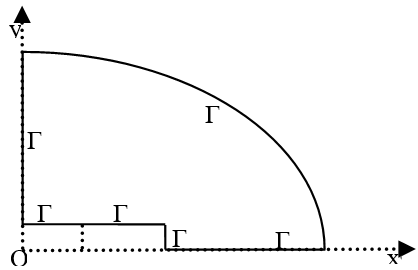Figure 1. Simplified model of the temperature field of surrounding rock and boundary conditions in coalface

According to the previous analysis, the boundary conditions of the temperature field of surrounding rock in coalface are as follows:

$\left\{\begin{array}{c}\left.\lambda \frac{\partial T}{\partial x}\right|_{\Gamma_{1}}=\alpha\left(T_{\Gamma_{1}}-T_{f}\right) \\ \left.\lambda \frac{\partial T}{\partial y}\right|_{\Gamma_{2}}=\alpha\left(T_{\Gamma_{2}}-T_{f}\right) \\ \left.\lambda \frac{\partial T}{\partial y}\right|_{\Gamma_{3}}=0 \\ \left.\lambda \frac{\partial T}{\partial x}\right|_{\Gamma_{4}}=0 \\ \left.\lambda \frac{\partial T}{\partial y}\right|_{\Gamma_{5}}=0 \\ \left.T\right|_{\Gamma_{6}}=T_{g u}\end{array}\right.$     (2)

where TΓ1 and TΓ2 are the wall temperatures of surrounding rock on boundaries Γ1 and Γ2 respectively ℃; λ is the heat conductivity coefficient of surrounding rock W/m·℃; α is the thermal conductivity of surrounding rock W/m2·℃; Tf is the air temperature in roadway surrounding rock ℃; Tgu is the initial temperature of surrounding rock ℃.

(3) The dimensionless treatment of the mathematical model of surrounding rock temperature field.

Some dimensionless parameters are introduced to improve the universality of calculation results. Moreover, the relationship between the unsteady heat exchange coefficient and the dimensionless parameters is analyzed. Then the mathematical model of the temperature field of surrounding rock in coalface is made non-dimensional by introducing the dimensionless parameters as follows : the Biot coefficient $B_{i}=\frac{\alpha h_{2}}{\lambda}$; the Peclet coefficient $P_{e}=\frac{v h_{2}}{a}$; the unsteady heat exchange coefficient $k_{u \tau}=\frac{k_{\tau} h_{2}}{\lambda}$; the dimensionless temperature of the surrounding rock $\theta=\frac{T-T_{f}}{T_{g u}-T_{f}}$; the dimensionless horizontal ordinate $X=\frac{x}{h_{2}}$; the dimensionless vertical coordinate $Y=\frac{y}{h_{2}}$; where h2 is half the mining height m; kτ is the unsteady heat exchange coefficient W/m2·℃; other symbols ibid.

Therefore, equation (1) can be deformed as:

$\frac{\partial^{2} \theta}{\partial X^{2}}+\frac{\partial^{2} \theta}{\partial Y^{2}}+P_{e} \frac{\partial \theta}{\partial X}=0$    (3)

Similarly, equation (2) is deformed as:

$\left\{\begin{array}{c}\left.\frac{\partial \theta}{\partial X}\right|_{\Gamma_{1}}=\left.B_{i} \theta\right|_{\Gamma_{1}} \\ \left.\frac{\partial \theta}{\partial Y}\right|_{\Gamma_{2}}=\left.B_{i} \theta\right|_{\Gamma_{2}} \\ \left.\frac{\partial \theta}{\partial Y}\right|_{\Gamma_{3}}=0 \\ \left.\frac{\partial \theta}{\partial X}\right|_{\Gamma_{4}}=0 \\ \left.\frac{\partial \theta}{\partial Y}\right|_{\Gamma_{5}}=0 \\ \left.\theta\right|_{\Gamma_{6}}=1\end{array}\right.$   (4)

The simultaneous differential equations composed of Equations (3) and (4) are the mathematical description of the temperature field of surrounding rock in the moving coordinate system. The analysis shows that the dimensionless temperature of surrounding rock θ and the unstable heat transfer criterion kare both the function of the Biot coefficient Bi, the Peclet coefficient Pe and the coalface geometry size s/h2.

2.2 The discretization of dimensionless the mathematical model of surrounding rock temperature field with finite volume method

(1) Meshing of the computational area of surrounding rock temperature field.

With triangles as the basic unit, the computational area of the temperature field of surrounding rock is divided with the grid size increasing in an equal proportion from wall surface to the deep rock in. In addition, the grid density is appropriately increased on the working space surface as shown in Figure 2 [18-19].

## 2.jpg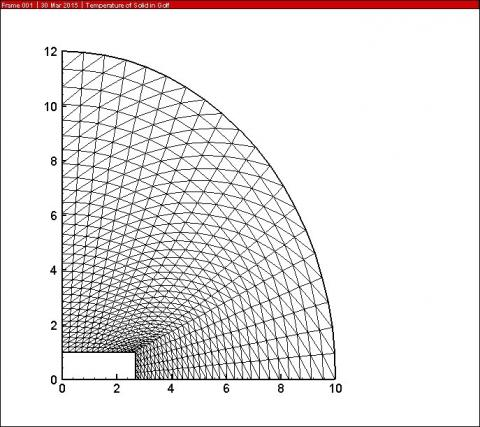Figure 2. Meshing of the computational area of surrounding rock temperature field

(2) The application of the finite volume method.

According to the basic theory of the finite volume method, the integral of control volume is used to discretize the equations. The differential equation is integrated in the computational area. By using the Green Formula, the calculation of surface integral is converted to curvilinear integral computation, and the integral equation is deduced as :

$\oint_{\Gamma} \frac{\partial \theta}{\partial X} d Y-\oint_{\Gamma} \frac{\partial \theta}{\partial Y} d X+\oint_{\Gamma} P_{e} \theta d Y=0$    (5)

## 3.jpg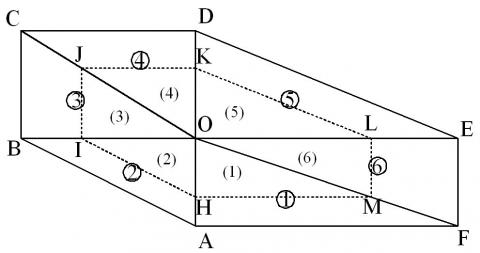Figure 3. Control volume of an internal node 

Any internal node O is analyzed and the hexagon ABCDEF is taken as the research object. As shown in Figure 3, the polygon HIJKLM composed of 6 triangles is the control volume of node O, in which equation (5) can be dispersed as :

$\sum_{k=1}^{6}\left(\frac{\partial \theta}{\partial X} \Delta Y-\frac{\partial \theta}{\partial Y} \Delta X\right)+\sum_{k=1}^{6} P e \theta \Delta Y=0$     (6)

where ΔX and ΔY are the projected length on X-axis and Y-axis of the k-th control volume of node O respectively.

Equation (6) can be abbreviated as:

$\sum_{k=1}^{n} Q_{l}=0$     (7)

Equation (6) or Equation (7) indicates that the energy equation deduced on any node can be deformed to the sum of several parts. Each part is determined by relative parameters of the node, or each unit related to the node making a contribution to the energy equation. Thus, the contribution of every related unit to the energy equation can be calculated. With the application of temperature interpolation function, the contribution of an internal triangle unit to its three nodes i, j and m can be deduced to the general formula:

$\left\{\begin{array}{l}Q_{i} \\ Q_{j} \\ Q_{m}\end{array}\right\}=\left[\begin{array}{ccc}k_{i i} & k_{i j} & k_{i m} \\ k_{j i} & k_{j j} & k_{j m} \\ k_{m i} & k_{m j} & k_{m m}\end{array}\right]\left\{\begin{array}{c}\theta_{i} \\ \theta_{j} \\ \theta_{m}\end{array}\right\}$     (8)

$k_{l l}=-\frac{1}{3 S}\left(b_{l}^{2}+c_{l}^{2}\right)-\frac{10}{27} P_{e} b_{l}$

$k_{l n}=k_{n l}=-\frac{1}{3 S}\left(b_{l} b_{n}+c_{l} c_{n}\right)-\frac{4}{27} P_{e} b_{l}$

$(l, n=i, j, m ; l \neq n)$

In the same way, the contribution of a boundary unit to each of its nodes can be calculated. If nodes i and j are both on the third boundary, the contribution of the boundary unit to nodes i and j can be deduced to the general formula:

$\left\{\begin{array}{l}Q_{i} \\ Q_{j}\end{array}\right\}=\left[\begin{array}{ll}u_{i i} & u_{i j} \\ u_{j i} & u_{j j}\end{array}\right]\left\{\begin{array}{l}\theta_{i} \\ \theta_{j}\end{array}\right\}$      (9)

$u_{i i}=u_{j j}=-\frac{10}{27} B_{i} \sqrt{b_{j}^{2}+c_{j}^{2}}$

$u_{i j}=u_{j i}=-\frac{4}{27} B_{i} \sqrt{b_{j}^{2}+c_{j}^{2}}$

The contribution equation is deduced at any node in the computational area, and all equations can be combined to a system of linear equations. The Gaussian elimination method is used to calculate the corresponding coefficient matrix, and the dimensionless temperature of each node can be obtained.

2.3 Design of the calculation program of the surrounding rock temperature field

Based on the calculation model deduced with the finite volume method and relative boundary conditions, a computer program is edited to calculate the temperature field of surrounding rock in coalface. The flow chart of calculating program is as shown in Figure 4.

## 4.png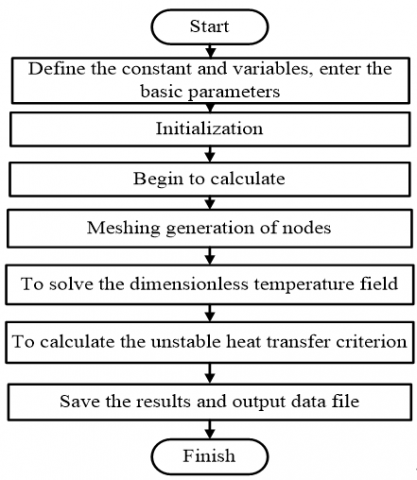Figure 4. Flow chart of calculating program of temperature field

3. Results

(1) When the ratio of face width and semi mining height is 8/3 for example, the dimensionless temperature distribution of surrounding rock is obtained by assuming both the Biot coefficient and Peclet coefficient to be parallel.

## 5.jpg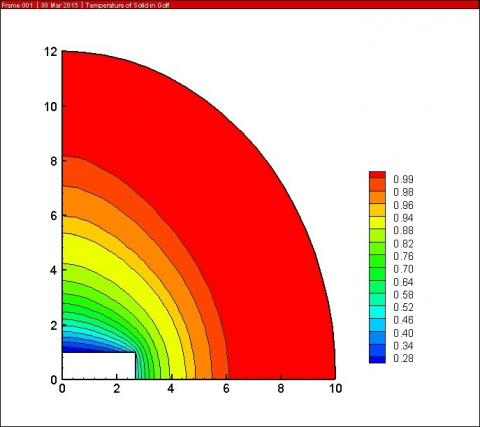Figure 5. Distribution of the dimensionless temperature of surrounding rock in coalface

As shown in Figure 5, the scope of the cooling zone in front of the working face is less than that along the roof and floor while the temperature gradient and wall temperature are both greater than that of the roof and floor.

(2) The dimensionless temperature along face width and height of the wall surface of surrounding rock is unequal. Taking the coalface with the ratio of face width and semi mining height is 8/3 for example; the dimensionless temperature distribution of wall surface of surrounding rock is obtained by assuming both the Biot coefficient and Peclet coefficient to be variants.

## 6.png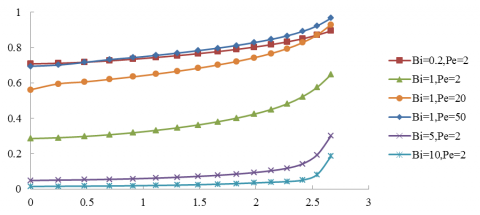Figure 6. Distribution of the dimensionless temperature of coal wall along face width

## 7.pngFigure 7. Distribution of the dimensionless temperature of coal wall along face height

As shown in Figures 6 and 7, the closer the coal wall, the greater the dimensionless temperature along the face width, while the closer the roof, the greater the dimensionless temperature along the coal wall. But the temperature variation of the coal wall is small along the face height. The dimensionless temperature of the coal wall increases with the increase of Peclet coefficient at a constant Biot coefficient, while the temperature decreases with the increase of Biot coefficient at a constant Peclet coefficient. Under the same condition, the greater the Biot coefficient and the more intensive the heat convection between the coal wall and air flow, the lower the dimensionless temperature of the coal wall.

Analysis of the dimensionless parameters introduced above indicates that the unstable heat transfer criterion is equal to the product of dimensionless temperature on the wall surface and the Biot coefficient. Therefore, the change rule of unstable heat transfer criterion can be deduced from the dimensionless temperature variation of wall surface. According to the dimensionless temperature variation, the closer the coal wall, the greater the unstable heat transfer criterion along the face width in the same circumstance. Furthermore, the unstable heat transfer criterion increases with the increase of Peclet coefficient at a constant Biot coefficient.

(3) When the ratio of face width and semi mining height is 1, 2, 3, 4 and 5, the temperature field of surrounding rock in coalface is calculated with different values of Biot coefficient and Peclet coefficient respectively. Furthermore, the unstable heat transfer criterion kunder different combinations of the above three parameters is obtained respectively. Each variation curve of unstable heat transfer criterion with increasing Peclet coefficient is obtained with different values of the Biot coefficient as shown in Figure 8 (a-e).

## 8a.png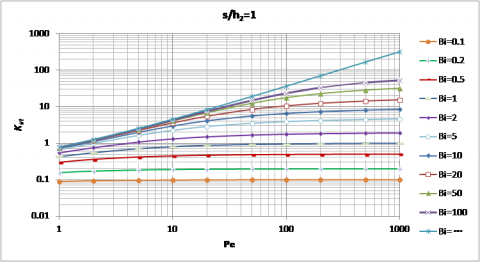(a)

## 8b.png(b)

## 8c.png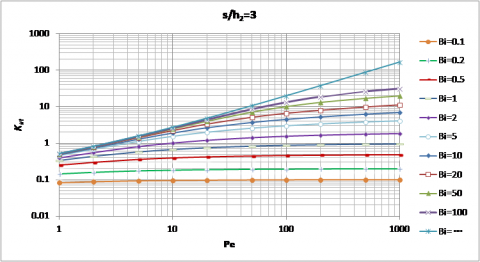(c)

## 8d.png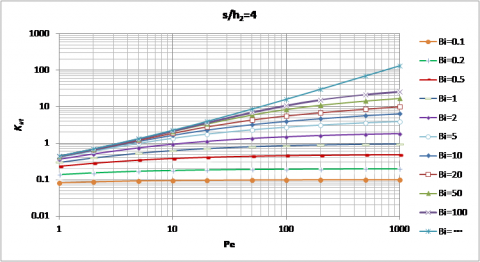(d)

## 8e.png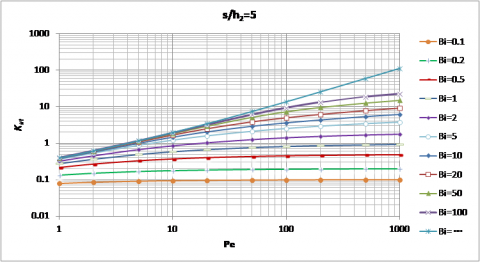(e)

Figure 8. The unstable heat transfer criterion of surrounding rock with different values of s/h2, Bi and Pe

As shown in Figure 8, no matter what the coalface geometrical size is, the unstable heat transfer criterion increases with the increase of Biot coefficient and Peclet coefficient.

Thus for any specific coalface, the values of Bi, Pe and s/h2 can be calculated first, and the unstable heat transfer criterion can be determined accordingly by the three parameters in Figure 8. Then the unsteady heat exchange coefficient between surrounding rock and air flow is obtained. When the calculated parameters are between some two legend values, the corresponding unstable heat transfer criterion can be obtained using the interpolation method. Therefore, based on much calculated data, the unsteady heat exchange coefficient between surrounding rock and air flow in coalface can be determined quickly and reliably by a consulting graph.

4. Conclusions

(1) By introducing the moving coordinate system, the unsteady heat conduction problem is converted to a steady state one. Thus, the complex calculation of heat dissipating capacity is greatly simplified.

(2) By carrying out the dimensionless analysis on coalfaces which have some commonalities, the universality of calculation results is improved. Thus, the results are given universal significance.

(3) With the finite volume method, the temperature field of surrounding rock in coalface is calculated and the dimensionless temperature distribution of surrounding rock is obtained. When the Biot coefficient and Peclet coefficient are assumed to be variants, the results reveal that the dimensionless temperature of the coal wall increases with the increase of Peclet coefficient at a constant Biot coefficient, while the temperature decreases with the increase of Biot coefficient at a constant Peclet coefficient. The relation curves of unstable heat transfer criterion with geometrical size, Biot coefficient and Peclet coefficient are obtained, which reveal that the unstable heat transfer criterion increases with the increase of Biot coefficient and Peclet coefficient.

(4) According to the relational curves of unstable heat transfer criterion with geometrical size, Biot coefficient and Peclet coefficient, the unstable heat transfer criterion can be determined quickly and reliably by a consulting graph for the mine cooling system designers and field engineering technicians. Based on it, the heat dissipating capacity of surrounding rock can be calculated, and this method provides critical parameters for forecasting air temperature and calculating chilling requirements in coalface.

Acknowledgment

This work was supported by the National Natural Science Foundation of China under the project No. 51174211.

References

1. S. Xin, Prevention and Cure of Thermal Hazard in  Mines (ed.), Introduction, chap. 1, China Coal Industry Publishing House, Beijing, 2011.

2. H. C. Yu, Mine Geothermal and Thermal Damage Control (ed.), Heat Sources in Mines, chap. 17, China Coal Industry Publishing House, Beijing, 1991.

3. R. L. Yan, and X. W. Hou, Mine Air Conditioning Technology (ed.), Prediction of the Thermodynamic Status Parameters of Mine Air, chap. 6, China Coal Industry Publishing House, Beijing, 1994.

4. A. H. Шербань, Mine Cooling Guide (ed.), Heat Transfer Coefficient, Unsteady  HeatTtransfer Coefficient and Moisture Exchange Coefficient,  chap. 13, China Coal Industry Publishing House, Beijing, 1982.

5. G. Danko and D. Bahrami,  Application  of MULTIFLUX for Air, Heat and Moisture Flow Simulations Proc. Symp. 12th U.S. / North American Mine Ventilation Symposium, pp. 267-274, 2008.

6. A. M. Starfield and A. L. Bleloch,  A New Method for the Computation of Heat and Moisture Transfer in a Partly Wet Airway, Journal of the South Africa Institute of Mining and Metallurgy, vol. 83, pp. 263-269, 1983.

7. A. M. Starfield, The Computation of Temperature Increases in Wet and Dry Airways, Journal of the Mine Ventilation Society of South Africa, vol. 19, pp. 157-165, 1966.

8. A. M. Starfield and A. J. Dickson, A Study of Heat Transfer and Moisture Pick-up in Mine  Airways, Journal of the South Africa Institute of Mining and Metallurgy, vol. 67, pp. 211-234, 1967.

9. I. S. Lowndes, A. J. Crossley and Z. Y. Yang, The Ventilation and Climate Modelling of Rapid Development Tunnel Drivages, Tunnelling and Underground Space Technology, vol. 19, pp. 139-150, 2004. DOI: 10.1016/j.tust.2003.09.003.

10. I. S. Lowndes, Z. Y. Yang, S. Jobling and C. Yates, A Parametric Analysis of a Tunnel  Climatic  Prediction and Planning Model, Tunnelling and Underground Space Technology, vol. 21, pp. 520-532, 2006. DOI: 10.1016/j.tust.2005.08.012.

11. Y. M. Wang, Y. Zhu, J. T. Li and F. C. Wang, Study on Heat Transfer between Country-rock and Airflow  in Deep Tunnels, Journal of China Coal Society, vol. 4, pp. 17-29, 1987. DOI: 10.13225 /j.cnki.jccs.1987.04.002.

12. J. L. Gao, W. Xu and X. B. Zhang, Treatment of Water Evaporation during Calculation of Temperature and Humidity of Airflow Caused by Heat Release from Surrounding Rock, Journal of China Coal Society, vol. 32(6), pp. 28-32, 2010. DOI: 10.13225/j.cnki.jccs.2010.06.026.

13. X. H. Zhou, J. R. Wang, G. B. Lu, Y. F. Shan and D. Liang, The Numerical Simulation of  Distribution  Law of Temperature Field in Coal Face, Journal of China Coal Society, vol. 27(1), pp. 59-63, 2002. DOI: 10.13225/j.cnki.jccs.2002.01.013.

14. P. D. Sun, Visualization Simulation of Temperature Distributions in Geothermal Field of Surrounding Rock of Deep Mine Tunnels, Rock and Soil Mechanics, vol.  10 supp. 2, pp. 222-226, 2005.

15. Y. P. Qin, F. H. Qin and H. Z. Dang, Computing the Unsteady-state Heat Transfer Criterion between Air and Rock Surrounding Airway with Finite Difference Method, Journal of Xiangtan Mining Institute,  vol. 13(1), pp. 6-10, 1998.

16. Y. P. Qin, H. Z. Dang and A. M. Liu, Calculation of  Heat Quantity from Rocks around Roadways with Boundary Element Method, Journal of China University of Mining and Technology, vol. 29(4), pp. 403-406, 2000.

17. Q. Wu, Y. P. Qin, A. M. Liu and Y. Q. Chen, Study on Temperature Field of Surrounding Rock with BEM, Journal of China University of Mining and Technology, vol. 11(1), pp. 6-9, 2001.

18. Y. P. Qin, F. H. Qin and M. X. Yu, The Study of Heat Transfer in Rock Surrounding Coal Face with Finite Element Method, Journal of Liaoning Technical University (Natural Science), vol. 18(4), pp. 342-346, 1999.

19. Q. Wu, Y. P. Qin, L. Guo and Q. Y. Wu, Calculation of the Heat Emitting from the Wall Rock at Drifting Face with Finite Element Method, China Safety Science Journal, vol. 12(6), pp. 33-36, 2001.

20. Y. P. Qin, H. T. Song, J. S. Wu and Z. Y. Dong, Numerical Analysis of Temperature Field  of Surrounding Rock under Periodic Boundary  Using Finite Volume Method, Journal of China Coal Society, vol. 40(7), pp. 1541-1549, 2015. DOI: 10.13225/j.cnki.jccs.2014.1432.

21. S. N. Singh, Numerical Study of Combined Natural Convection, Conduction and Surface Radiation Heat Transfer in Open Top, Side Vented Cavities, International Journal of Heat and Technology, vol. 26(2), pp. 101-109, 2008.

22. M. Y. Kim, Assessment of the Axisymmetric Radiative Heat Transfer in a Cylindrical Enclosure with the Finite Volume Method, International Journal of Heat and Mass Transfer, vol. 51, pp. 5144-5153, 2008. DOI: 10.1016/j.ijheatmasstransfer.2008.03.012.

23. W. B. Li, Y. M. Wu, H. L. Fu and J. B. Zhang, Long- term Continuous In-situ Monitoring of Tunnel Lining Surface Temperature in Cold Region and its Application, International Journal of Heat and Technology, vol. 33(2) pp. 39-44, 2015. DOI: 10.18280/ijht.330206.

24. J. Shao, W. Q. Sun, A. R. He and B. Li, Research on the Thermal Calculation Model  of  High  Strength Aluminum Strip Rolling Based on Finite Difference Method, International Journal of Heat and Technology, vol. 33(1), pp. 91-98, 2015. DOI: 10.18280/ijht.330113.

25. Y. P. Qin, H. Z. Dang and F. Qu,  Dimensionless Analysis on Heat Dissipation of Rock Surrounding in Coal Face, Journal of China Coal Society, vol. 23(1), pp. 62-66, 1998. DOI: 10.13225/j.cnki.jccs.1998.01.013.

26. Y. H. Wang, Study on Simulation of Spontaneous Combustion in Goaf on the Moving Coordinate with Finite Volume Method, Ph.D. thesis, China  University of Mining and Technology (Beijing), Haidian District, Beijing, 2009.

27. W. Liu, H. T. Song and X. F. Li,  Dimensionless Analysis on Gas Emission Law around Tunneling Face, Journal of China Coal Society, vol. 40(4), pp. 882-887, 2015. DOI: 10.13225/j.cnki.jccs.2014.3024.